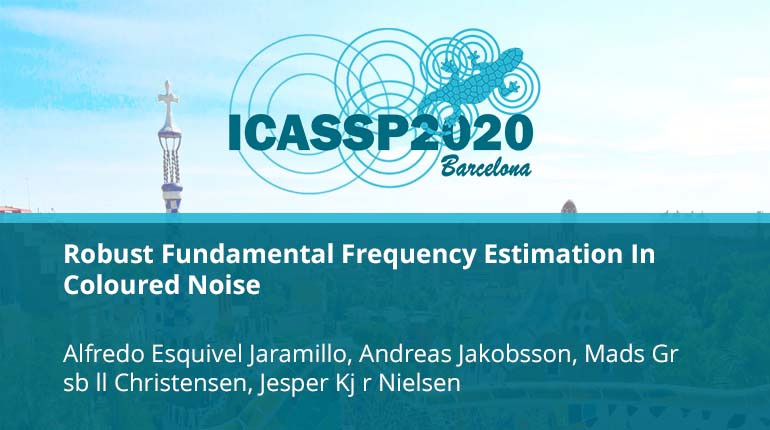# Robust Fundamental Frequency Estimation In Coloured Noise

Collection:Most parametric fundamental frequency estimators make the implicit assumption that any corrupting noise is additive, white Gaussian. Under this assumption, the maximum likelihood (ML) and the least squares estimators are the same, and statistically effici
• IEEE MemberUS \$11.00
• Society MemberUS \$0.00
• IEEE Student MemberUS \$11.00
• Non-IEEE MemberUS \$15.00
Purchase

## Robust Fundamental Frequency Estimation In Coloured Noise

Most parametric fundamental frequency estimators make the implicit assumption that any corrupting noise is additive, white Gaussian. Under this assumption, the maximum likelihood (ML) and the least squares estimators are the same, and statistically effici